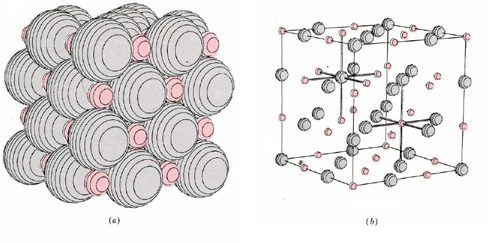# 6.5: Ions and Noble-Gas Electron Configurations

$$\newcommand{\vecs}{\overset { \rightharpoonup} {\mathbf{#1}} }$$ $$\newcommand{\vecd}{\overset{-\!-\!\rightharpoonup}{\vphantom{a}\smash {#1}}}$$$$\newcommand{\id}{\mathrm{id}}$$ $$\newcommand{\Span}{\mathrm{span}}$$ $$\newcommand{\kernel}{\mathrm{null}\,}$$ $$\newcommand{\range}{\mathrm{range}\,}$$ $$\newcommand{\RealPart}{\mathrm{Re}}$$ $$\newcommand{\ImaginaryPart}{\mathrm{Im}}$$ $$\newcommand{\Argument}{\mathrm{Arg}}$$ $$\newcommand{\norm}{\| #1 \|}$$ $$\newcommand{\inner}{\langle #1, #2 \rangle}$$ $$\newcommand{\Span}{\mathrm{span}}$$ $$\newcommand{\id}{\mathrm{id}}$$ $$\newcommand{\Span}{\mathrm{span}}$$ $$\newcommand{\kernel}{\mathrm{null}\,}$$ $$\newcommand{\range}{\mathrm{range}\,}$$ $$\newcommand{\RealPart}{\mathrm{Re}}$$ $$\newcommand{\ImaginaryPart}{\mathrm{Im}}$$ $$\newcommand{\Argument}{\mathrm{Arg}}$$ $$\newcommand{\norm}{\| #1 \|}$$ $$\newcommand{\inner}{\langle #1, #2 \rangle}$$ $$\newcommand{\Span}{\mathrm{span}}$$$$\newcommand{\AA}{\unicode[.8,0]{x212B}}$$

When considering the formation of LiH, one aspect deserves explanation. If the transfer of one electron from Li to H is energetically favorable, why is the same not true for the transfer of a second electron to produce Li2+H2– ? Certainly the double charges on Li2+ and H2– would attract more strongly than the single charges on Li+ and H, and the doubly charged ions would be held more tightly in the crystal lattice. The answer to this question can be found by looking back at the diagram below.Figure 6.5.1 The image above shows electron density diagrams for Lithium, Hydrogen, and the ionic compound LiH. Next to each element is the electron orbital diagram, which shows the symbolic form of the electron density diagram.

Removal of a second electron from Li would require much more energy than the removal of the first because this second electron would be a 1s (from the smaller circle in the image above, closer to the nucleus) electron rather than a 2s electron. Not only is this second electron much closer to the nucleus, but it also is very poorly shielded from the nucleus, meaning it's attraction to the nucleus is strong. It is not surprising, therefore, that the second ionization energy of Li (the energy required to remove this second electron) is 7297 kJ mol–1 almost 14 times as large as the first ionization energy! Such a colossal energy requirement is enough to insure that only the outermost electron (the valence electron) of Li will be removed and that the inner 1s kernel with its helium-type electron configuration will remain intact.

A similar argument applies to the acceptance of a second electron by the H atom to form the H2– ion. If such an ion were to be formed, the extra electron would have to occupy the 2s orbital (outside the mix of red and grey dots of the H- ion pictured above). Its electron cloud would extend far from the nucleus (even farther than for the 2s electron in Li, because the nuclear charge in H2– would only be +1, as opposed to +3 in Li), and it would be quite high in energy. So much energy would be needed to force a second electron to move around the H nucleus in this way, that only one electron is transferred. The ion formed has the formula H and a helium-type 1s2 electronic structure instead of an H2- ion with a 1s22s1 electronic structure.

The simple example of lithium hydride is typical of all ionic compounds which can be formed by combination of two elements. Invariably we find that one of the two elements has a relatively low ionization energy and is capable of easily losing one or more electrons. The other element has a relatively high electron affinity and is able to accept one or more electrons into its structure. The ions formed by this transfer of electrons almost always have an electronic structure which is the same as that of noble gas, and all electrons are paired in each ion. The resulting compound is always a solid in which the ions are arranged in a three-dimensional array or crystal lattice similar to, though not always identical with, that shown in the LiH crystal lattice below.In such a solid the nearest neighbors of each anion are always cations and vice versa, and the solid is held together by the forces of attraction between the ions of opposite sign. An everyday example of such an ionic compound is ordinary table salt, sodium chloride, whose formula is NaCl. As we shall see in the next section, sodium is an element with a low ionization energy, and chlorine is an element with a high electron affinity.

On the microscopic level crystals of sodium chloride consist of an array of sodium ions, Na+, and chloride ions, Cl, packed together in a lattice like that shown for lithium hydride. The chloride ions are chlorine atoms which have gained an electron and thus have the electronic structure 1s22s22p63s23p6, the same as that of the noble-gas argon. The sodium ions are sodium atoms which have lost an electron, giving them the structure 1s22s22p6, the same as that of the noble-gas neon. All electrons in both kinds of ions are paired.